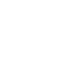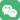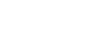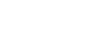2018工程技术领域欧洲大学排行400-010-8000

# 2018工程技术领域欧洲大学排行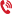咨询热线：17710313735 立即咨询
QS公布的2018工程技术领域欧洲大学排名，意大利两所知名工科院校表现非常抢眼，米兰理工大学排名欧洲第六，仅次于剑桥大学、苏黎世联邦理工学院、帝国理工学院、牛津大学与洛桑联邦理工学院，排名超过了德国慕尼黑工业大学、荷兰代尔夫特理工大学、德国亚琛工业大学等欧洲一流工科院校！想留学，没方向？还不点我快来聊？2018 工程技术领域欧洲大学排行 -- 米兰理工与都灵理工排名前十

QS 公布的 2018 工程技术领域欧洲大学排名，意大利两所知名工科院校表现非常抢眼，米兰理工大学排名欧洲第六，仅次于剑桥大学、苏黎世联邦理工学院、帝国理工学院、牛津大学与洛桑联邦理工学院，排名超过了德国慕尼黑工业大学、荷兰代尔夫特理工大学、德国亚琛工业大学等欧洲一流工科院校！

1           3             剑桥大学                    英国

2           4             苏黎世联邦理工学院     瑞士

3           6             帝国理工学院               英国

4 =        8              牛津大学                    英国

5          12             洛桑联邦理工学院         瑞士

6 =       17             米兰理工大学              意大利

7 =       22             代尔夫特理工大学          荷兰

8          25               慕尼黑工业大学           德国

9 =       31              亚琛工业大学               德国

10 =      33               都灵理工大学              意大利

11 =      39               柏林工业大学              德国

12 =      41                皇家理工大学              瑞典

13 =      51                卡尔斯鲁厄理工学院     德国

14 =      53                  丹麦技术大学             丹麦

14 =      53                 曼彻斯特大学              英国

16 =      58                 伦敦大学学院              英国

17 =      64                    鲁汶大学                比利时

18 =      73          巴黎中央理工 - 高等电力学院 法国

18 =       73               爱丁堡大学                    英国

20 =       76               巴黎综合理工学院           法国

20 =        76              莫斯科国立大学             俄罗斯

20 =        76                马德里理工大学            西班牙

23 =         81                 奥尔堡大学                 丹麦

24 =         81              加泰罗尼亚理工大学      西班牙

25 =         89               圣三一学院                 爱尔兰

26             92               斯图加特大学                德国

27 =          95             达姆斯塔特工业大学         德国

28 =          97              德累斯顿工业大学            德国

30 =         100               罗马大学                     意大利

30 =         100            博洛尼亚大学                  意大利

32 =          103           埃因霍温理工大学              荷兰

32 =          103             都柏林大学                    爱尔兰

34 =          106            南安普顿大学                     英国

35 =          113             奥尔胡斯大学                    丹麦

35 =           113           查尔姆斯理工大学               瑞典

37 =           119             伯明翰大学                      英国

37 =            119           布里斯托大学                    英国

39 =            121            谢菲尔德大学                   英国

40 =            124           雅典国立工业大学             希腊

40 =             124            利兹大学                        英国

42                127            巴塞罗那大学                  西班牙

43 =             128                帕多瓦大学                 意大利

44 =             131            慕尼黑大学                       德国

44 =             131                隆德大学                      瑞典

46                 133              诺丁汉大学                     英国

47                 134            瓦伦西亚理工大学              西班牙

48 =              135               伦敦国王学院                 英国

48 =              135               波尔图大学                   葡萄牙

50 =              137                华威大学                     英国

50 =              137               里斯本大学                  葡萄牙还没看够？点我点我！专业顾问等你来“撩”~# 相关推荐

• 粉丝102
• 文章361### 相关顾问